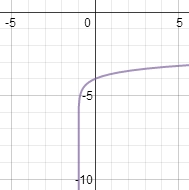# How to Graph Logarithms: Transformations and Effects on Domain/Range

Instructions:

Choose an answer and hit 'next'. You will receive your score and answers at the end.

question 1 of 3

### Which direction will the graph of this equation be shifted? y = log (x + 2)

Create Your Account To Take This Quiz

As a member, you'll also get unlimited access to over 75,000 lessons in math, English, science, history, and more. Plus, get practice tests, quizzes, and personalized coaching to help you succeed.

Try it risk-free for 30 days. Cancel anytime.

### 2. Which equation represents this graph?Create your account to access this entire worksheet
A Premium account gives you access to all lesson, practice exams, quizzes & worksheets
Quizzes, practice exams & worksheets
Certificate of Completion
Create an account to get started

There are two methods for graphing logarithms. You can use these materials to review how to properly graph these equations and how to make graphs shift on the coordinate plane.

## Quiz & Worksheet Goals

Use this quiz and worksheet to test how well you understand these concepts:

• Graphing a logarithm
• Using a logarithm equation to shift a graph
• Graphing transformations
• Domain and range

## Skills Practiced

Use these materials to practice the following:

• Problem solving - use acquired knowledge to solve logarithm graphing practice problems
• Knowledge application - use your knowledge to answer questions about transformations
• Making connections - use understanding of the concept of domain and range

If you want a more in-depth look at graphing logarithms, check out the accompanying video lesson called How to Graph Logarithms: Transformations and Effects on Domain/Range. In that lesson you will cover these objectives:

• Define logarithmic equations
• Review the definition of exponentials
• Show how to graph a basic logarithm formula
• Provide tactics for graphing logarithms with additional transformations
• Discuss how transformations affect domain and range
Final ExamMath 103: Precalculus
Status: Not Started
Chapter ExamEvaluating Exponential and Logarithmic Functions
Status: Not Started

Support